# MP Board Class 6th Maths Solutions Chapter 10 Mensuration Ex 10.1

## MP Board Class 6th Maths Solutions Chapter 10 Mensuration Ex 10.1

Question 1.
Find the perimeter of each of the following figures: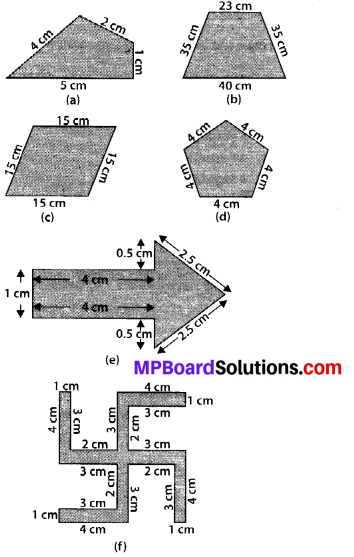Solution:
(a) Perimeter = Sum of all the sides
= 4 cm + 2 cm + 1 cm + 5 cm = 12 cm

(b) Perimeter = Sum of all the sides
= 23 cm + 35cm + 40 cm + 35cm = 133 cm

(c) Perimeter = Sum of all the sides
= 15 cm + 15 cm + 15 cm + 15 cm = 60 cm

(d) Perimeter = Sum of all the sides
= 4 cm + 4 cm + 4 cm + 4 cm + 4 cm = 20 cm

(e) Perimeter = Sum of all the sides
= 1 cm + 4 cm + 0.5 cm + 2.5 cm + 2.5 cm + 0.5 cm + 4 cm = 15 cm

(f) Perimeter = Sum of all the sides
= 4 cm + 1 cm + 3 cm + 2 cm + 3 cm + 4 cm + 1 cm + 3 cm + 2 cm + 3 cm + 4 cm + 1 cm + 3 cm + 2 cm + 3 cm + 4 cm + 1 cm + 3 cm + 2 cm + 3 cm = 52 cm

Question 2.
The lid of a rectangular box of sides 40 cm by 10 cm is sealed all round with tape. What is the length of the tape required?
Solution:
Total length of tape required
= Perimeter of rectangle
= 2 (length + breadth)
= 2 (40 +10) cm = 2 × 50 cm = 100 cm = 1 m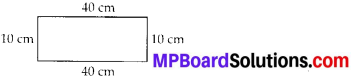Thus, the total length of tape required is 100 cm or 1 m.

Question 3.
A table-top measures 2 m 25 cm by 1 m 50 cm. What is the perimeter of the table-top?
Solution:
Length of table-top = 2 m 25 cm = 2.25 m
Breadth of table-top = 1 m 50 cm = 1.50 m
Perimeter of table-top = 2 × (length + breadth)
= 2 × (2.25 + 1.50) m
= 2 × 3.75 m = 7.50 m
Thus, perimeter of table-top is 7.5 m.Question 4.
What is the length of the wooden strip required to frame a photograph of length and breadth 32 cm and 21 cm respectively?
Solution:
Length of wooden strip
= Perimeter of photograph
= 2 × (length + breadth)
= 2 (32 + 21) cm = 2 × 53 cm = 106 cm
Thus, the length of the wooden strip required is 106 cm.

Question 5.
A rectangular piece of land measures 0.7 km by 0.5 km. Each side is to be fenced with 4 rows of wires. What is the length of the wire needed?
Solution:
Since 4 rows of wires are needed. Therefore, the total length of wire is equal to 4 times the perimeter of land.
Perimeter of land = 2 × (length + breadth)
= 2 × (0.7 + 0.5) km = 2 × 1.2 km = 2.4 km
= 2.4 × 1000 m = 2400 m
Thus, the length of wire
= 4 × 2400 m = 9600 m = 9.6 km

Question 6.
Find the perimeter of each of the following shapes:
(a) A triangle of sides 3 cm, 4 cm and 5 cm.
(b) An equilateral triangle of side 9 cm.
(c) An isosceles triangle with equal sides 8 cm each and third side 6 cm.
Solution:
(a) Perimeter of ∆ABC
= AB + BC + CA
= 3 cm+ 5 cm+ 4 cm
= 12 cm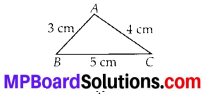(b) Perimeter of equilateral ∆ABC
= 3 × side
= 3 × 9 cm
= 27 cm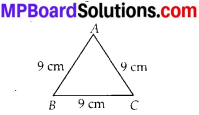(c) Perimeter of ∆ABC
= AB + BC + CA
= 8 cm + 6 cm + 8 cm
= 22 cm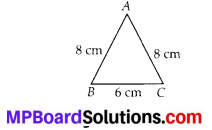Question 7.
Find the perimeter of a triangle with sides measuring 10 cm, 14 cm and 15 cm.
Solution:
Perimeter of triangle
= Sum of all three sides
= 10 cm + 14 cm + 15 cm = 39 cm
Thus, perimeter of triangle is 39 cm.Question 8.
Find the perimeter of a regular hexagon with each side measuring 8 m.
Solution:
Perimeter of a regular hexagon
= 6 × length of one side
= 6 × 8m
= 48m
Thus, the perimeter of the regular hexagon is 48 m.

Question 9.
Find the side of the square whose perimeter is 20 m.
Solution:
Perimeter of square = 4 × side
⇒ 20 m = 4 × side ⇒ side = $$\frac{20}{4}$$ m = 5m
Thus, the side of square is 5 m.

Question 10.
The perimeter of a regular pentagon is 100 cm. How long is its each side?
Solution:
Perimeter of regular pentagon = 5 × side
⇒ 100 cm = 5 × side ⇒ side = $$\frac{100}{5}$$ cm = 20 cm
Thus, the side of regular pentagon is 20 cm.Question 11.
A piece of string is 30 cm long. What will be the length of each side if the string is used to form:
(a) a square?
(b) an equilateral triangle?
(c) a regular hexagon?
Solution:
Length of string = Perimeter of each shape
(a) Perimeter of square = 4 × side
⇒ 30 cm = 4 × side ⇒ side = $$\frac{30}{4}$$ cm = 7.5 cm
Thus, the length of each side of square will be 7.5 cm.

(b) Perimeter of equilateral triangle = 3 × side
⇒ 30 cm = 3 × side ⇒ side = $$\frac{30}{3}$$ cm = 10 cm
Thus, the length of each side of equilateral triangle will be 10 cm.

(c) Perimeter of regular hexagon = 6 × side
⇒ 30 cm = 6 × side ⇒ side = $$\frac{30}{6}$$ cm = 5 cm
Thus, the length of each side of regular hexagon will be 5 cm.

Question 12.
Two sides of a triangle are 12 cm and 14 cm. The perimeter of the triangle is 36 cm. What is its third side?
Solution:
Let the length of third side be x cm. Length of other two sides are 12 cm and 14 cm.
Now, perimeter of triangle = 36 cm
⇒ 12 + 14 + x = 36 ⇒ 26 + x = 36
⇒ x = 36 – 26 ⇒ x = 10
Thus, the length of third side is 10 cm.

Question 13.
Find the cost of fencing a square park of side 250 m at the rate of Rs. 20 per metre.
Solution:
Side of square park = 250 m
Perimeter of square park = 4 × side
= 4 × 250 m = 1000 m
Since, cost of fencing for 1 metre = Rs. 20
Therefore, cost of fencing for 1000 metres
= Rs. 20 × 1000 = Rs. 20,000

Question 14.
Find the cost of fencing a rectangular park of length 175 m and breadth 125 m at the rate of Rs. 12 per metre.
Solution:
Length of rectangular park = 175 m
Breadth of rectangular park = 125 m
Perimeter of park = 2 × (length + breadth)
= 2 × (175 + 125) m = 2 × 300 m = 600 m
Since, cost of fencing park for 1 metre = Rs. 12
Therefore, cost of fencing park for 600 m = Rs. 12 × 600 = Rs. 7,200

Question 15.
Sweety runs around a square park of side 75 m. Bulbul runs around a rectangular park with length 60 m and breadth 45 m. Who covers less distance?
Solution:
Distance covered by Sweety
= Perimeter of square park = 4 × side
= 4 × 75 m = 300 m
Thus, distance covered by Sweety is 300 m.
Now, distance covered by Bulbul
= Perimeter of rectangular park
= 2 × (length + breadth)
= 2 × (60 + 45) m = 2 × 105 m = 210 m
Thus, Bulbul covers a distance of 210 m.
So, Bulbul covers less distance.

Question 16.
What is the perimeter of each of the following figures? What do you infer from the answers?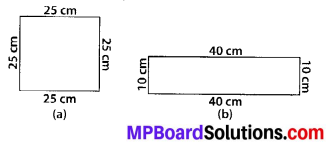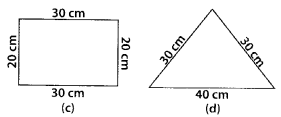Solution:
(a) Perimeter of square = 4 × side
= 4 × 25 cm = 100 cm

(b) Perimeter of rectangle
= 2 × (length + breadth)
= 2 × (40 + 10) cm = 2 × 50 cm = 100 cm

(c) Perimeter of rectangle
= 2 × (length + breadth)
= 2 × (30 + 20) cm = 2 × 50 cm = 100 cm

(d) Perimeter of triangle = Sum of all sides
= 30 cm + 30 cm + 40 cm
= 100 cm
Thus, all the figures have same perimeter.Question 17.
Avneet buys 9 square paving slabs, each with side of $$\frac{1}{2}$$ m. He lays them in the form of a square.
(a) What is the perimeter of his arrangement [see fig. (i)]?
(b) Shari does not like his arrangement. She gets him to lay them out like a cross. What is the perimeter of her arrangement [see fig. (ii)]?
(c) Which has a greater perimeter?
(d) Avneet wonders if there is a way of getting an even greater perimeter. Can you find a way of doing this? (The paving slabs must meet along complete edges i.e. they cannot be broken.)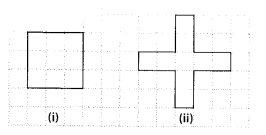Solution:
(a) Side of one small square = $$\frac{1}{2}$$ m
∴ Side of given square = $$\frac{3}{2}$$ m
Perimeter of square = 4 × side
= 4 × $$\frac{3}{2}$$ m = 6 m

(b) Perimeter of given figure
= Sum of all sides = 20 × $$\frac{1}{2}$$ m = 10 m

(c) The arrangement cross has greater perimeter.
(d) It is not possible to determine the arrangement with perimeter greater than 10 m.